# Building NLP Classifiers Cheaply With Transfer Learning and Weak Supervision

## A Step-by-Step Guide for Building an Anti-Semitic Tweet Classifier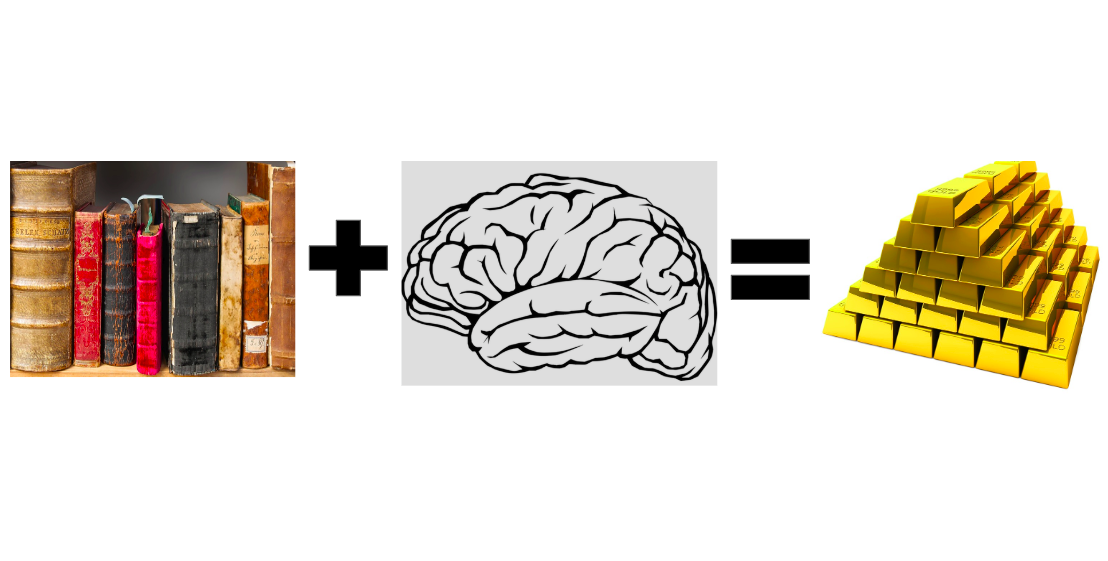Text + Intelligence = Gold… But, how can we mine it cheaply?

# Background

## Weak Supervision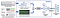Overview of the Data Programming Paradigm with Snorkel
`# Set voting values.ABSTAIN = 0 POSITIVE = 1 NEGATIVE = 2# Detects common conspiracy theories about jews owning the world.GLOBALISM = r"\b(Soros|Adelson|Rothschild|Khazar)"def jews_symbols_of_globalism(tweet_text):    return POSITIVE if re.search(GLOBALISM, tweet_text) else ABSTAIN`

## Transfer Learning and ULMFiT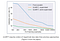ULMFiT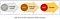Introduction to ULMFiT

# Step-By-Step Guide for Building an Anti-Semitic Tweet Classifier

## First step: Data Collection and Setting a Target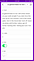View of Airtable for Text Labeling
`DATA_PATH = "../data"train = pd.read_csv(os.path.join(DATA_PATH, "train_tweets.csv"))test = pd.read_csv(os.path.join(DATA_PATH, "test_tweets.csv"))LF_set = pd.read_csv(os.path.join(DATA_PATH, "LF_tweets.csv"))train.shape, LF_set.shape, test.shape>> ((24738, 6), (733, 7), (438, 7))`

## Second Step: Building a Training Set With Snorkel

`# Common insults against jews.INSULTS = r"\bjew (bitch|shit|crow|fuck|rat|cockroach|ass|bast(a|e)rd)"def insults(tweet_text):    return POSITIVE if re.search(INSULTS, tweet_text) else ABSTAIN`
`# If tweet author is jewish then it's likely not anti-semitic.JEWISH_AUTHOR = r"((\bI am jew)|(\bas a jew)|(\bborn a jew)"def jewish_author(tweet_tweet):    return NEGATIVE if re.search(JEWISH_AUTHOR, tweet_tweet) else ABSTAIN`
`# We build a matrix of LF votes for each tweetLF_matrix = make_Ls_matrix(LF_set, LFs)# Get true labels for LF setY_LF_set = np.array(LF_set['label'])display(lf_summary(sparse.csr_matrix(LF_matrix),                    Y=Y_LF_set,                    lf_names=LF_names.values()))`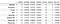LF Summary
`label_coverage(LF_matrix)>> 0.8062755798090041`
`from metal.label_model.baselines import MajorityLabelVotermv = MajorityLabelVoter()Y_train_majority_votes = mv.predict(LF_matrix)print(classification_report(Y_LFs, Y_train_majority_votes))`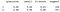Classification Report for Majority Voter Baseline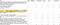Google Sheet I used for tuning my LFs
`Ls_train = make_Ls_matrix(train, LFs)# You can tune the learning rate and class balance.label_model = LabelModel(k=2, seed=123)label_model.train_model(Ls_train, n_epochs=2000, print_every=1000,                         lr=0.0001,                         class_balance=np.array([0.2, 0.8]))`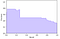Precision-Recall Curve for Label Model
`# To use all information possible when we fit our classifier, we can # actually combine our hand-labeled LF set with our training set.Y_train = label_model.predict(Ls_train) + Y_LF_set`

## Third Step: Build Classification Model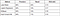Baselines
`data_lm = TextLMDataBunch.from_df(train_df=LM_TWEETS,         valid_df=df_test, path="")learn_lm = language_model_learner(data_lm, pretrained_model=URLs.WT103_1, drop_mult=0.5)`
`learn_lm.unfreeze()`
`for i in range(20):    learn_lm.fit_one_cycle(cyc_len=1, max_lr=1e-3, moms=(0.8, 0.7))    learn_lm.save('twitter_lm')`
`learn_lm.predict("i hate jews", n_words=10)>> 'i hate jews are additional for what hello you brother . xxmaj the'learn_lm.predict("jews", n_words=10)>> 'jews out there though probably okay jew back xxbos xxmaj my'`
`# Classifier model datadata_clas = TextClasDataBunch.from_df(path = "",                                       train_df = df_trn,                                      valid_df = df_val,                                                                               vocab=data_lm.train_ds.vocab,                                       bs=32,                                       label_cols=0)learn = text_classifier_learner(data_clas, drop_mult=0.5)learn.freeze()`
`learn.lr_find(start_lr=1e-8, end_lr=1e2)learn.recorder.plot()`
`learn.fit_one_cycle(cyc_len=1, max_lr=1e-3, moms=(0.8, 0.7))learn.freeze_to(-2)learn.fit_one_cycle(1, slice(1e-4,1e-2), moms=(0.8,0.7))learn.freeze_to(-3)learn.fit_one_cycle(1, slice(1e-5,5e-3), moms=(0.8,0.7))learn.unfreeze()learn.fit_one_cycle(4, slice(1e-5,1e-3), moms=(0.8,0.7))`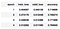A few training epochs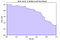Precision-Recall curve of ULMFiT with Weak Supervision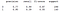Classification Report for ULMFiT Model

## Having Fun With Our Model

`learn.predict("george soros controls the government")>> (Category 1, tensor(1), tensor([0.4436, 0.5564]))learn.predict("george soros doesn't control the government")>> (Category 0, tensor(0), tensor([0.7151, 0.2849]))`
`learn.predict("fuck jews")>> (Category 1, tensor(1), tensor([0.1996, 0.8004]))learn.predict("dirty jews")>> (Category 1, tensor(1), tensor([0.4686, 0.5314]))`
`learn.predict("Wow. The shocking part is you're proud of offending every serious jew, mocking a religion and openly being an anti-semite.")>> (Category 0, tensor(0), tensor([0.9908, 0.0092]))`
`learn.predict("my cousin is a russian jew from ukraine- 💜🌻💜 i'm so glad they here")>> (Category 0, tensor(0), tensor([0.8076, 0.1924]))learn.predict("at least the stolen election got the temple jew shooter off the drudgereport. I ran out of tears.")>> (Category 0, tensor(0), tensor([0.9022, 0.0978]))`

## Does Weak Supervision Actually Help?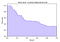Precision-Recall curve of ULMFiT without Weak Supervision

## Sculpt

Text Intelligence Without Coding

Written by

## Abraham Starosta

MS in CS from Stanford and Working on NLP — abraham@sculptintel.com

Text Intelligence Without Coding

Written by

## Abraham Starosta

MS in CS from Stanford and Working on NLP — abraham@sculptintel.com

## More From Medium

Medium is an open platform where 170 million readers come to find insightful and dynamic thinking. Here, expert and undiscovered voices alike dive into the heart of any topic and bring new ideas to the surface. Learn more

Follow the writers, publications, and topics that matter to you, and you’ll see them on your homepage and in your inbox. Explore

If you have a story to tell, knowledge to share, or a perspective to offer — welcome home. It’s easy and free to post your thinking on any topic. Write on Medium